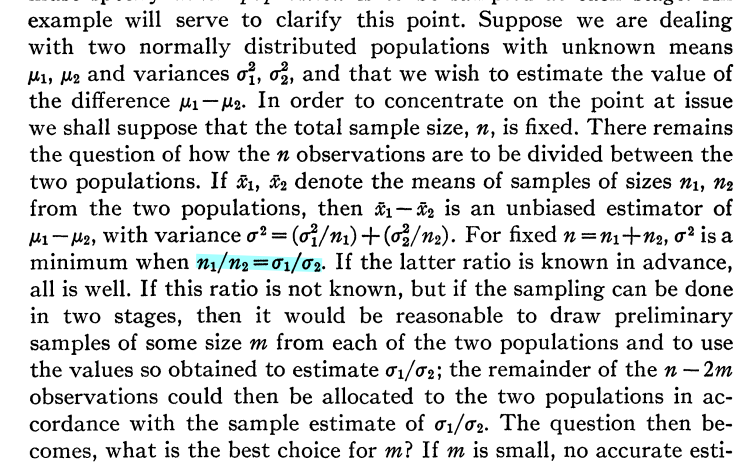# Resource Allocation for Statistical Tasks

A similar allocation task was discussed by Robbins (1952).Consider the following toy problem. There are two distributions P_1 = N(\mu_1, \sigma_1^2) and P_2 = N(\mu_2, \sigma_2^2), and we want to draw a total of n samples from the two distributions (i.e., n_1+n_2=n) such that the two empirical mean estimates \hat{\mu}_1 and \hat{\mu}_2 have roughly the same mean-squared error. What are the optimal choices of n_1 and n_2? It is easy to check that we must allocate n_i \propto \sigma_i^2.

If the two variances are not known, we must then develop an adaptive or active sampling strategy to estimate the variances, and use them to guide the allocation. Such problems, of learning the means of several distributions uniformly well in terms of mean-squared error, has been studied under the name of active learning in bandits. However, the existing methods are not applicable to problems beyond estimating the means.

## Learning Discrete Distributions and MDPs

In my ICML 2020 S. Shekhar, T. Javidi and M. Ghavamzadeh, “Adaptive Sampling for learning probability distributions,” ICML 2020.

paper, I considered the task of learning K discrete distributions in terms of general distance measures, such as total-variation, \ell_2^2, f-divergence, and separation metric.

The key observation was that, for all these distance measures, we can approximate the underlying task with a minimax problem with a convex-concave objective function. This observation allowed a unified treatment of the allocation problem for all the distance measures mentioned above. We proposed a general allocation scheme for this problem, and showed that it is also minimax near-optimal.

The J. Tarbouriech, S. Shekhar, M. Pirotta, M. Ghavamzadeh, and A. Lazaric, “Active model estimation in Markov decision processes,” UAI 2020.

ideas developed in the previous paper for learning distributions with i.i.d. observations can be exteneded to learn the transition strucutre of Markov Decision Processes (MDPs), as we showed in our UAI 2020 paper.

## Fair Dataset Construction

Consider the problem of training a single classifier (e.g., a handwriting recognition system) that must be deployed on inputs drawn from K populations (e.g., people from different age-groups). Since the task might be inherently harder for some groups (e.g., very young, or very old people), how should a dataset be constructed to compensate for this?

Building upon the insights from our previous works, in our NeurIPS 2021 paper, S. Shekhar, G. Fields, M. Ghavamzadeh and T. Javidi, “Adaptive sampling for minimax fair classification,” NeurIPS 2021.

I developed a practical adaptive dataset construction scheme that can be provably used to learn minimax fair classifiers. Empirical results with deep neural networks (DNNs) validated the benefits of representative datasets.